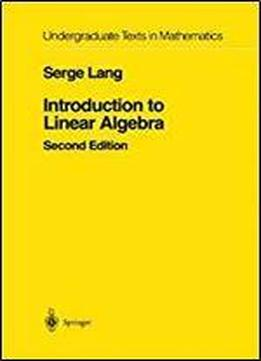Introduction To Linear Algebra (undergraduate Texts In Mathematics) 2nd Edition by Serge Lang / 1997 / English / PDF

This is a short text in linear algebra, intended for a one-term course. In the first chapter, Lang discusses the relation between the geometry and the algebra underlying the subject, and gives concrete examples of the notions which appear later in the book. He then starts with a discussion of linear equations, matrices and Gaussian elimination, and proceeds to discuss vector spaces, linear maps, scalar products, determinants, and eigenvalues. The book contains a large number of exercises, some of the routine computational type, while others are conceptual.

views: 429